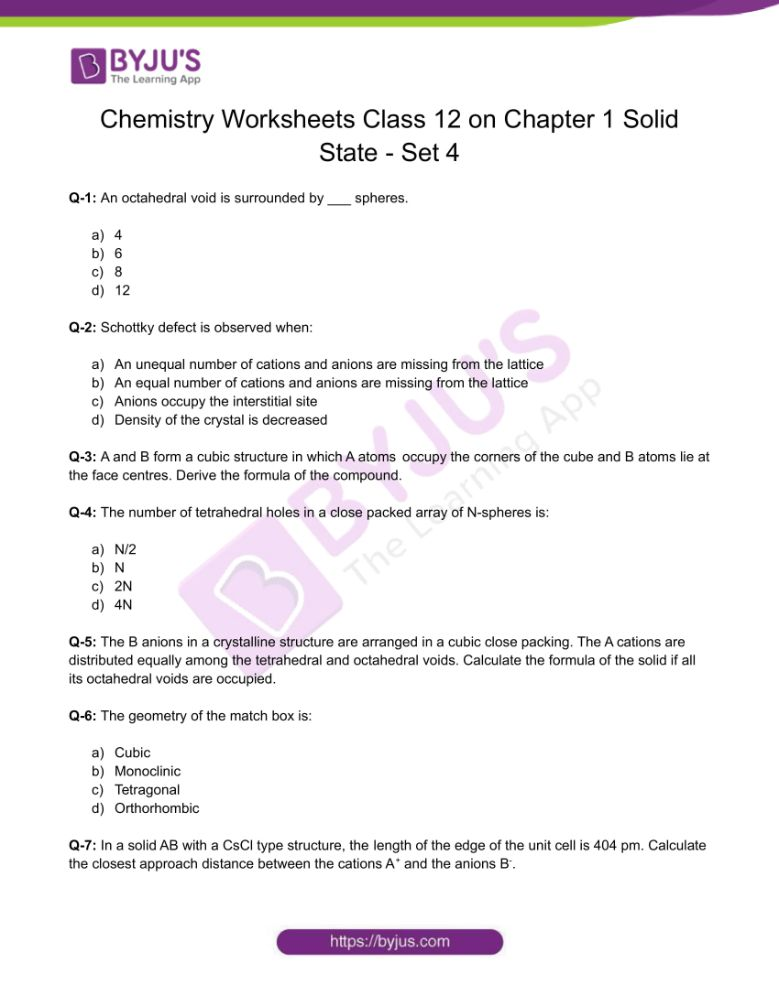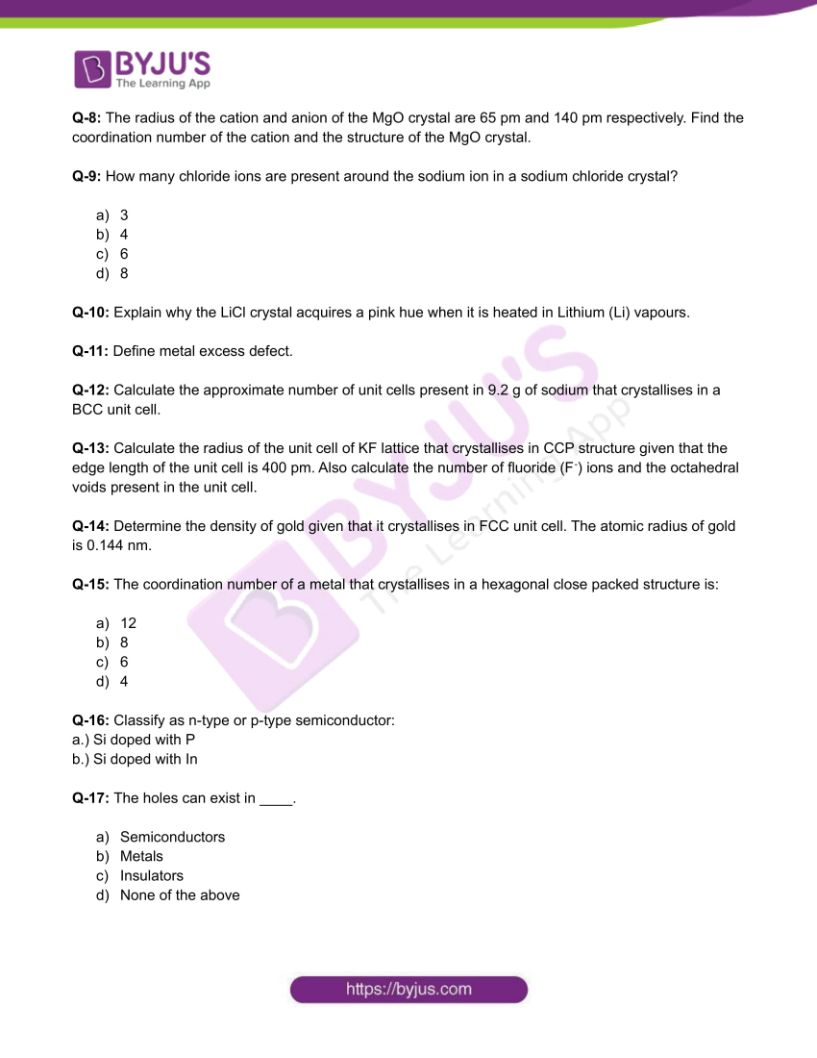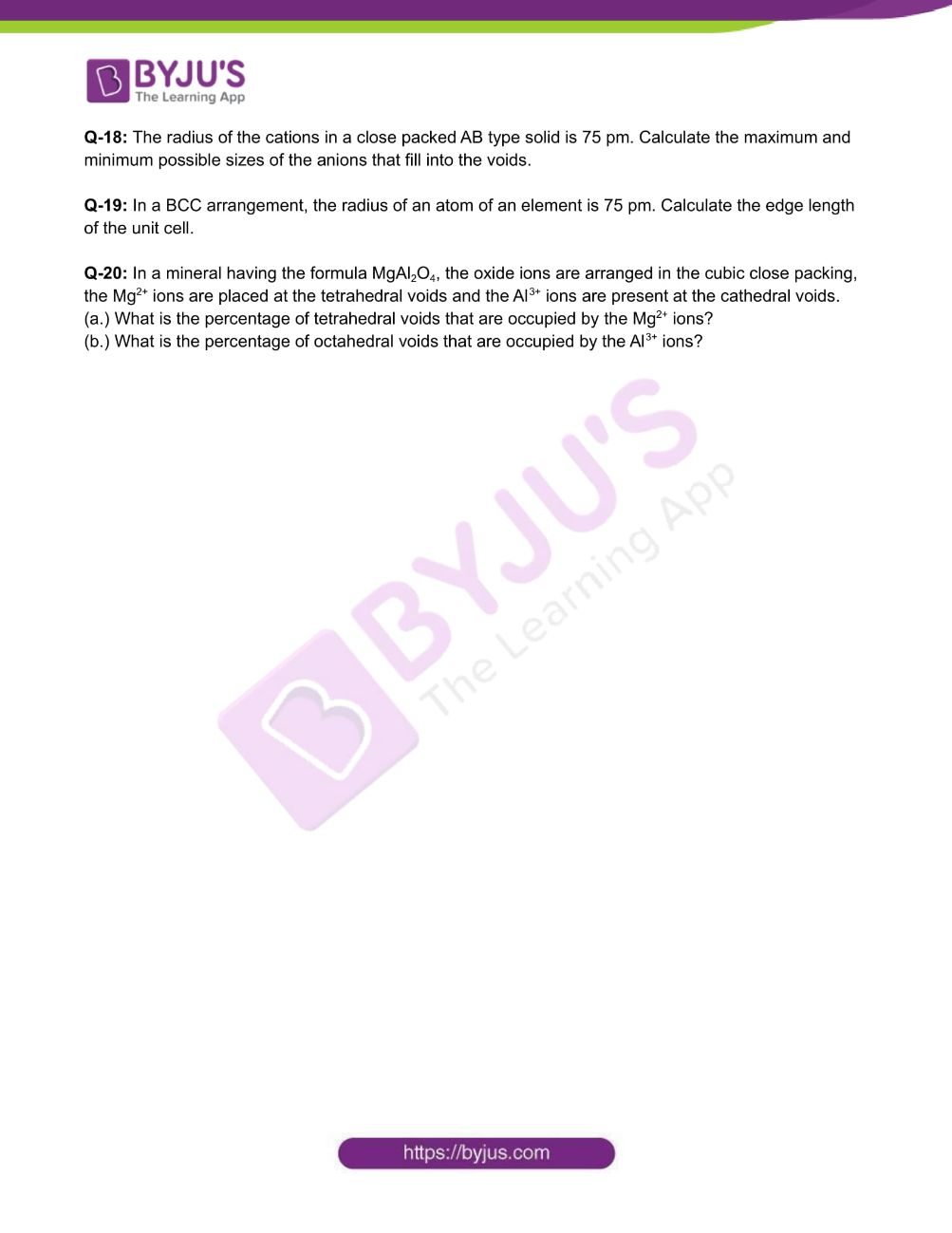Checkout JEE MAINS 2022 Question Paper Analysis : Checkout JEE MAINS 2022 Question Paper Analysis :

# Chemistry Worksheets Class 12 on Chapter 1 Solid State with Answers - Set 4

Solids are incompressible solid substances that mainly exist as – crystalline solids and amorphous solids. In the crystalline solids, the particles have a long range order which means that the particles are arranged in a regular fixed pattern in the three dimensional space. In amorphous solids, there is no regular fixed pattern in the three dimensional space and this gives the solids only the short range order of constituent particles.

## 👇## CBSE Class 12 Chemistry Chapter 1 Solid State Worksheet – Set 4

Q-1: An octahedral void is surrounded by ___ spheres.

a.) 4

b.) 6

c.) 8

d.) 12

Q-2: Schottky defect is observed when:

a.) An unequal number of cations (A+) and anions (B) are missing from the lattice

b.) An equal number of cations (A+) and anions (B) are missing from the lattice

c.) Anions (B) occupy the interstitial site

d.) Density of the crystal is decreased

Q-3: A and B form a cubic structure. In this structure, the A atoms lie at the corners of the cube and B atoms lie at the face centres. Derive the formula of the compound.

Q-4: The number of tetrahedral holes in a close packed array containing N-spheres is:

a.) N/2

b.) N

c.) 2N

d.) 4N

Q-5: The B anions in a crystalline structure are arranged in a cubic close packing. The A cations are distributed equally among the tetrahedral and octahedral voids. Calculate the formula of the solid if all its octahedral voids are occupied.

Q-6: The geometry of the match box is:

a.) Cubic

b.) Monoclinic

c.) Tetragonal

d.) Orthorhombic

Q-7: In a solid AB with a CsCl type structure, the length of the edge of the unit cell is 404 pm. Calculate the closest approach distance between the cations A+ and the anions B.

Q-8: The radius of the cation and anion of the MgO crystal are 65 pm and 140 pm respectively. Find the coordination number of the cation and the structure of the MgO crystal.

Q-9: How many chloride ions are present around the sodium ion in a sodium chloride crystal?

a.) 3

b.) 4

c.) 6

d.) 8

Q-10: Explain why the LiCl crystal acquires a pink hue when it is heated in Lithium (Li) vapours.

Q-11: Define metal excess defect.

Q-12: Calculate the approximate number of unit cells present in 9.2 g of sodium that crystallises in a BCC unit cell.

Q-13: Calculate the radius of the unit cell of KF lattice that crystallises in CCP structure given that the edge length of the unit cell is 400 pm. Also calculate the number of fluoride (F) ions and the octahedral voids present in the unit cell.

Q-14: Determine the density of gold given that it crystallises in FCC unit cell. The atomic radius of gold is 0.144 nm.

Q-15: The coordination number of a metal crystallising in a HCP structure is:

a.) 12

b.) 8

c.) 6

d.) 4

Q-16: Classify as n-type or p-type semiconductor:

a.) Si doped with P

b.) Si doped with In

Q-17: The holes can exist in ____.

a.) Semiconductors

b.) Metals

c.) Insulators

d.) None of the above

Q-18: The radius of the cations in a close packed AB type solid is 75 pm. Calculate the maximum and minimum possible sizes of the anions that fill into the voids.

Q-19: In a BCC arrangement, the radius of an atom of an element is 75 pm. Calculate the edge length of the unit cell.

Q-20: In a mineral having the formula MgAl2O4, the oxide ions are arranged in the cubic close packing, the Mg2+ ions are placed at the tetrahedral voids and the Al3+ ions are present at the cathedral voids.

(a.) What is the percentage of tetrahedral voids that are occupied by the Mg2+ ions?

(b.) What is the percentage of octahedral voids that are occupied by the Al3+ ions?

Download the PDF to access answers to the Chemistry Worksheet for Class 12 Chemistry Chapter 1 Solid State Set -4.